Chapter 4 Electric Current, Resistance, and Ohm’s Law

# 4.8 Resistors in Series and Parallel

### Summary

• Draw a circuit with resistors in parallel and in series.
• Calculate the voltage drop of a current across a resistor using Ohm’s law.
• Contrast the way total resistance is calculated for resistors in series and in parallel.
• Explain why total resistance of a parallel circuit is less than the smallest resistance of any of the resistors in that circuit.
• Calculate total resistance of a circuit that contains a mixture of resistors connected in series and in parallel.

Electric circuits are commonplace. Some are simple, such as those in flashlights. Others, such as those used in supercomputers, are extremely complex.

This collection of modules takes the topic of electric circuits a step beyond simple circuits. When the circuit is purely resistive, everything in this module applies to both DC and AC. Matters become more complex when capacitance is involved. We do consider what happens when capacitors are connected to DC voltage sources, but the interaction of capacitors and other non-resistive devices with AC is left for a later chapter. Finally, a number of important DC instruments, such as meters that measure voltage and current, are covered in this chapter.

Most circuits have more than one component, called a resistor that limits the flow of charge in the circuit. A measure of this limit on charge flow is called resistance. The simplest combinations of resistors are the series and parallel connections illustrated in Figure 1. The total resistance of a combination of resistors depends on both their individual values and how they are connected.

# Resistors in Series

When are resistors in series? Resistors are in series whenever the flow of charge, called the current, must flow through devices sequentially. For example, if current flows through a person holding a screwdriver and into the Earth, then R1 in Figure 1(a) could be the resistance of the screwdriver’s shaft, R2 the resistance of its handle, R3 the person’s body resistance, and R4 the resistance of her shoes.

Figure 2 shows resistors in series connected to a voltage source. It seems reasonable that the total resistance is the sum of the individual resistances, considering that the current has to pass through each resistor in sequence. (This fact would be an advantage to a person wishing to avoid an electrical shock, who could reduce the current by wearing high-resistance rubber-soled shoes. It could be a disadvantage if one of the resistances were a faulty high-resistance cord to an appliance that would reduce the operating current.)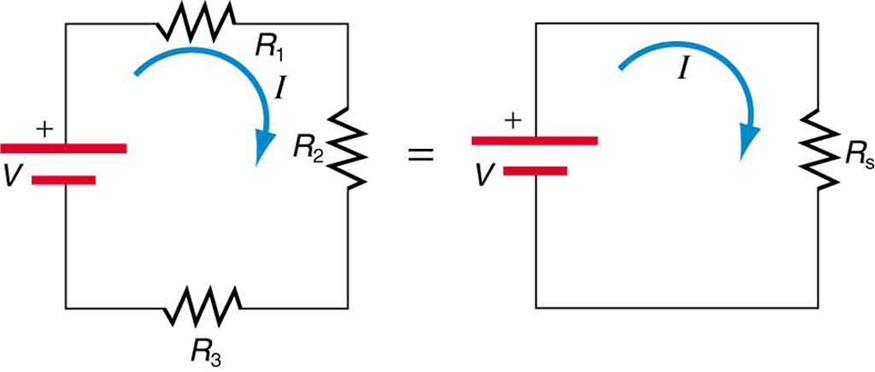Figure 2. Three resistors connected in series to a battery (left) and the equivalent single or series resistance (right).

To verify that resistances in series do indeed add, let us consider the loss of electrical power, called a voltage drop, in each resistor in Figure 2.

According to Ohm’s law, the voltage drop, V, across a resistor when a current flows through it is calculated using the equation V=IR, where I equals the current in amps (A) and R is the resistance in ohms Ω. Another way to think of this is that is the voltage necessary to make a current  flow through a resistance R.

So the voltage drop across R1 is  V1 = IR that across R is V= IR  and that across Ris V= IR . The sum of these voltages equals the voltage output of the source; that is,

V = V1 + V2 + V3
This equation is based on the conservation of energy and conservation of charge. Electrical potential energy can be described by the equation PE = qV, where q is the electric charge and V is the voltage. Thus the energy supplied by the source is qV, while that dissipated by the resistors is
qV = qV1 + qV2 + qV3

### Connections: Conservation Laws

The derivations of the expressions for series and parallel resistance are based on the laws of conservation of energy and conservation of charge, which state that total charge and total energy are constant in any process. These two laws are directly involved in all electrical phenomena and will be invoked repeatedly to explain both specific effects and the general behaviour of electricity.

These energies must be equal, because there is no other source and no other destination for energy in the circuit. Thus, qV = qV1 + qV2 + qV3. The charge cancels, yielding V = V1 + V2 + V3, as stated. (Note that the same amount of charge passes through the battery and each resistor in a given amount of time, since there is no capacitance to store charge, there is no place for charge to leak, and charge is conserved.)

Now substituting the values for the individual voltages gives

V = IR1 + IR2 + IR3  = I (R1 + R2 + R)

Note that for the equivalent single series resistance Rs we have

V = IRs

This implies that the total or equivalent series resistance Rs of three resistors is

Rseries = Rs = R1 + R2 + R

This logic is valid in general for any number of resistors in series; thus, the total resistance Rs of a series connection is

Rseries = Rs = R1 + R2 + R + ……

as proposed. Since all of the current must pass through each resistor, it experiences the resistance of each, and resistances in series simply add up.

### Example 1: Calculating Resistance, Current, Voltage Drop, and Power Dissipation: Analysis of a Series Circuit

Suppose the voltage output of the battery in Figure 2 is 12.0 V, and the resistances are  R1 = 1.00 Ω , R2 = 6.00Ω, and R3 = 13.0 Ω. (a) What is the total resistance? (b) Find the current. (c) Calculate the voltage drop in each resistor, and show these add to equal the voltage output of the source. (d) Calculate the power dissipated by each resistor. (e) Find the power output of the source, and show that it equals the total power dissipated by the resistors.

Strategy and Solution for (a)

The total resistance is simply the sum of the individual resistances, as given by this equation:

Rs = R1 + R2 + R3 = 1.00  Ω + 6.00 Ω + 13.0  Ω = 20.0 Ω

Strategy and Solution for (b)

The current is found using Ohm’s law, V = IR. Entering the value of the applied voltage and the total resistance yields the current for the circuit: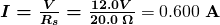Strategy and Solution for (c)

The voltage—or IR drop—in a resistor is given by Ohm’s law. Entering the current and the value of the first resistance yields

V1 = IR1 = (0.600 A)(1.0 Ω) = 0.600 V

Similarly,

V2 = IR2 = (0.600 A)(6.0 Ω) = 3.60 V

and

V3 = IR3 = (0.600 A)(13.0Ω) = 7.80 V

Discussion for (c)

The three  IR  drops add to 12.0 V, as predicted:

V1 + V2 + V3 = (0.600 + 3.60 + 7.80) V  = 12.0 V

Strategy and Solution for (d)

The easiest way to calculate power in watts (W) dissipated by a resistor in a DC circuit is to use Joule’s law, P = IV, where P is electric power. In this case, each resistor has the same full current flowing through it. By substituting Ohm’s law V = IR  into Joule’s law, we get the power dissipated by the first resistor as

P1 = I2 R1 = (0.600 A)(1.00 Ω) = 0.360 W

Similarly,

P2 = I2 R2 = (0.600 A)(6.00 Ω) = 2.16 W

and

P3 = I2 R3 = (0.600 A)(13.0 Ω) = 4.68 W

Discussion for (d)

Power can also be calculated using either P = IV  or P = V2/R, where is the voltage drop across the resistor (not the full voltage of the source). The same values will be obtained.

Strategy and Solution for (e)

The easiest way to calculate power output of the source is to use P=IV, where V is the source voltage. This gives

P = (0.600 A)(12.0 V) = 7.20 W

Discussion for (e)

Note, coincidentally, that the total power dissipated by the resistors is also 7.20 W, the same as the power put out by the source. That is,

P1 + P2 + P3 = (0.360 + 2.16 + 4.68) W = 7.20 W.

Power is energy per unit time (watts), and so conservation of energy requires the power output of the source to be equal to the total power dissipated by the resistors.

### Major Features of Resistors in Series

1. Series resistances add: Rs = R1 + R2 + R3 + …..
2. The same current flows through each resistor in series.
3. Individual resistors in series do not get the total source voltage, but divide it.

# Resistors in Parallel

Figure 3 shows resistors in parallel, wired to a voltage source. Resistors are in parallel when each resistor is connected directly to the voltage source by connecting wires having negligible resistance. Each resistor thus has the full voltage of the source applied to it.

Each resistor draws the same current it would if it alone were connected to the voltage source (provided the voltage source is not overloaded). For example, an automobile’s headlights, radio, and so on, are wired in parallel, so that they utilize the full voltage of the source and can operate completely independently. The same is true in your house, or any building. (See Figure 3(b).)Figure 3. (a) Three resistors connected in parallel to a battery and the equivalent single or parallel resistance. (b) Electrical power setup in a house. (credit: Dmitry G, Wikimedia Commons)

To find an expression for the equivalent parallel resistance Rp, let us consider the currents that flow and how they are related to resistance. Since each resistor in the circuit has the full voltage, the currents flowing through the individual resistors are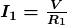,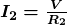, and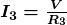. Conservation of charge implies that the total current I produced by the source is the sum of these currents:

I = I1 + I2 + I3

Substituting the expressions for the individual currents gives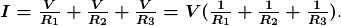Note that Ohm’s law for the equivalent single resistance gives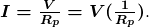The terms inside the parentheses in the last two equations must be equal. Generalizing to any number of resistors, the total resistance Rp of a parallel connection is related to the individual resistances by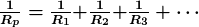This relationship results in a total resistance  Rp that is less than the smallest of the individual resistances. (This is seen in the next example.) When resistors are connected in parallel, more current flows from the source than would flow for any of them individually, and so the total resistance is lower.

### Example 2: Calculating Resistance, Current, Power Dissipation, and Power Output: Analysis of a Parallel Circuit

Let the voltage output of the battery and resistances in the parallel connection in Figure 3 be the same as the previously considered series connection: V = 12.0 V,  R1 = 1.00 Ω, R2 = 6.00 Ω, and R3 = 13.0 Ω. (a) What is the total resistance? (b) Find the total current. (c) Calculate the currents in each resistor, and show these add to equal the total current output of the source. (d) Calculate the power dissipated by each resistor. (e) Find the power output of the source, and show that it equals the total power dissipated by the resistors.

Strategy and Solution for (a)

The total resistance for a parallel combination of resistors is found using the equation below. Entering known values gives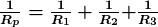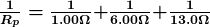Thus,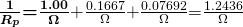(Note that in these calculations, each intermediate answer is shown with an extra digit.)

We must invert this to find the total resistance Rp. This yields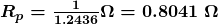The total resistance with the correct number of significant digits is  Rp = 0.804 Ω.

Discussion for (a)

R is, as predicted, less than the smallest individual resistance.

Strategy and Solution for (b)

The total current can be found from Ohm’s law, substituting Rp for the total resistance. This gives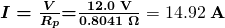Discussion for (b)

Current for each device is much larger than for the same devices connected in series (see the previous example). A circuit with parallel connections has a smaller total resistance than the resistors connected in series.

Strategy and Solution for (c)

The individual currents are easily calculated from Ohm’s law, since each resistor gets the full voltage. Thus,

I = V / R1  = (12.0 V) / (1.00 Ω) = 12.0 A

Similarly,

I = V / R2  = (12.0 V) / (6.00 Ω) = 2.00 A

and

I = V / R3  = (12.0 V) / (13.0 Ω) = 0.92 A

Discussion for (c)

The total current is the sum of the individual currents:

I1 + I2 + I3 = 14.92 A

This is consistent with conservation of charge.

Strategy and Solution for (d)

The power dissipated by each resistor can be found using any of the equations relating power to current, voltage, and resistance, since all three are known. Let us use  P = V2/R  since each resistor gets full voltage. Thus,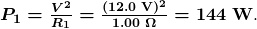Similarly,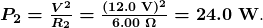and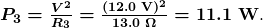Discussion for (d)

The power dissipated by each resistor is considerably higher in parallel than when connected in series to the same voltage source.

Strategy and Solution for (e)

The total power can also be calculated in several ways. Choosing P = IV , and entering the total current, yields

P = IV = (14.92 A)(12.0 V) = 179 W.

Discussion for (e)

Total power dissipated by the resistors is also 179 W:

P1 + P2 + P3 = 144 W  + 24.0 W  + 11.1 W = 179 W.

This is consistent with the law of conservation of energy.

Overall Discussion

Note that both the currents and powers in parallel connections are greater than for the same devices in series.

### Major Features of Resistors in Parallel

1. Parallel resistance is found from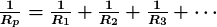, and it is smaller than any individual resistance in the combination.
2. Each resistor in parallel has the same full voltage of the source applied to it. (Power distribution systems most often use parallel connections to supply the myriad devices served with the same voltage and to allow them to operate independently.)
3. Parallel resistors do not each get the total current; they divide it.

# Combinations of Series and Parallel

More complex connections of resistors are sometimes just combinations of series and parallel. These are commonly encountered, especially when wire resistance is considered. In that case, wire resistance is in series with other resistances that are in parallel.

Combinations of series and parallel can be reduced to a single equivalent resistance using the technique illustrated in Figure 4. Various parts are identified as either series or parallel, reduced to their equivalents, and further reduced until a single resistance is left. The process is more time consuming than difficult.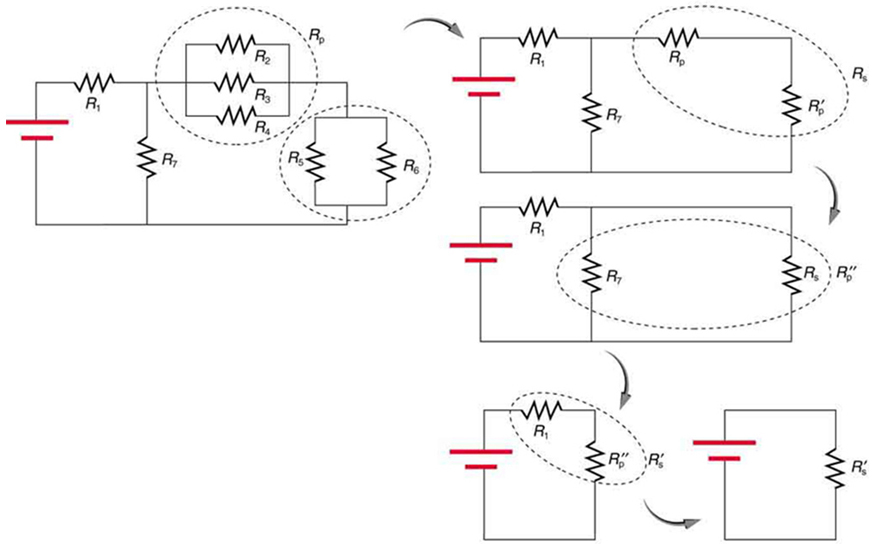Figure 4. This combination of seven resistors has both series and parallel parts. Each is identified and reduced to an equivalent resistance, and these are further reduced until a single equivalent resistance is reached.

The simplest combination of series and parallel resistance, shown in Figure 5, is also the most instructive, since it is found in many applications. For example, R1 could be the resistance of wires from a car battery to its electrical devices, which are in parallel.  R2 and R3  could be the starter motor and a passenger compartment light. We have previously assumed that wire resistance is negligible, but, when it is not, it has important effects, as the next example indicates.

### Example 3: Calculating Resistance, IR Drop, Current, and Power Dissipation: Combining Series and Parallel Circuits

Figure 5 shows the resistors from the previous two examples wired in a different way—a combination of series and parallel. We can consider R1 to be the resistance of wires leading to R2 and R3. (a) Find the total resistance. (b) What is the IR drop in R1? (c) Find the current I2 through R2. (d) What power is dissipated by R2?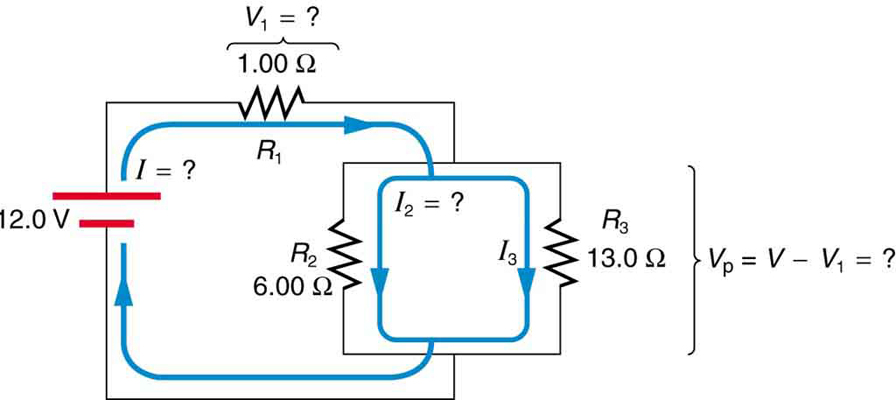Figure 5. These three resistors are connected to a voltage source so that R2 and R3 are in parallel with one another and that combination is in series with R1.

Strategy and Solution for (a)

To find the total resistance, we note that R2 and R3 are in parallel and their combination Rp is in series with R1. Thus the total (equivalent) resistance of this combination is

Rtotal = R1 + Rp

First, we find Rp using the equation for resistors in parallel and entering known values: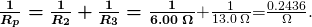Inverting gives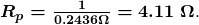So the total resistance is

Rtot = R1 + Rp = 1.00  Ω+ 4.11 Ω= 5.11 Ω .

Discussion for (a)

The total resistance of this combination is intermediate between the pure series and pure parallel values 20.0 Ω and 0.804 Ω , respectively) found for the same resistors in the two previous examples.

Strategy and Solution for (b)

To find the IR  drop in R1, we note that the full current I flows through R1. Thus its IR drop is

V1 = I R

We must find I before we can calculate V1 The total current is found using Ohm’s law for the circuit. That is,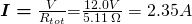Entering this into the expression above, we get

V1 = IR1 = ( 2.35 A )(1.00 Ω) = 2.35 V

Discussion for (b)

The voltage applied to Rand R3 is less than the total voltage by an amount V1. When wire resistance is large, it can significantly affect the operation of the devices represented by R2 and R3.

Strategy and Solution for (c)

To find the current through R2, we must first find the voltage applied to it. We call this voltage V, because it is applied to a parallel combination of resistors. The voltage applied to both R2 and R3 is reduced by the amount V1, and so it is

V = V – V1 = 12.0 V – 2.34 V = 9.65 V

Now the current I2 through resistance R2 is found using Ohm’s law: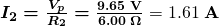Discussion for (c)

The current is less than the 2.00 A that flowed through R2 when it was connected in parallel to the battery in the previous parallel circuit example.

Strategy and Solution for (d)

The power dissipated by R2 is given by

P = (I2)2 R2 = (1.61 A)(6.00 Ω) = 15.5 W

Discussion for (d)

The power is less than the 24.0 W this resistor dissipated when connected in parallel to the 12.0-V source.

# Practical Implications

One implication of this last example is that resistance in wires reduces the current and power delivered to a resistor. If wire resistance is relatively large, as in a worn (or a very long) extension cord, then this loss can be significant. If a large current is drawn, the IR  drop in the wires can also be significant.

For example, when you are rummaging in the refrigerator and the motor comes on, the refrigerator light dims momentarily. Similarly, you can see the passenger compartment light dim when you start the engine of your car (although this may be due to resistance inside the battery itself).

What is happening in these high-current situations is illustrated in Figure 6. The device represented by Rhas a very low resistance, and so when it is switched on, a large current flows. This increased current causes a larger IR  drop in the wires represented by R1, reducing the voltage across the light bulb (which is R2), which then dims noticeably.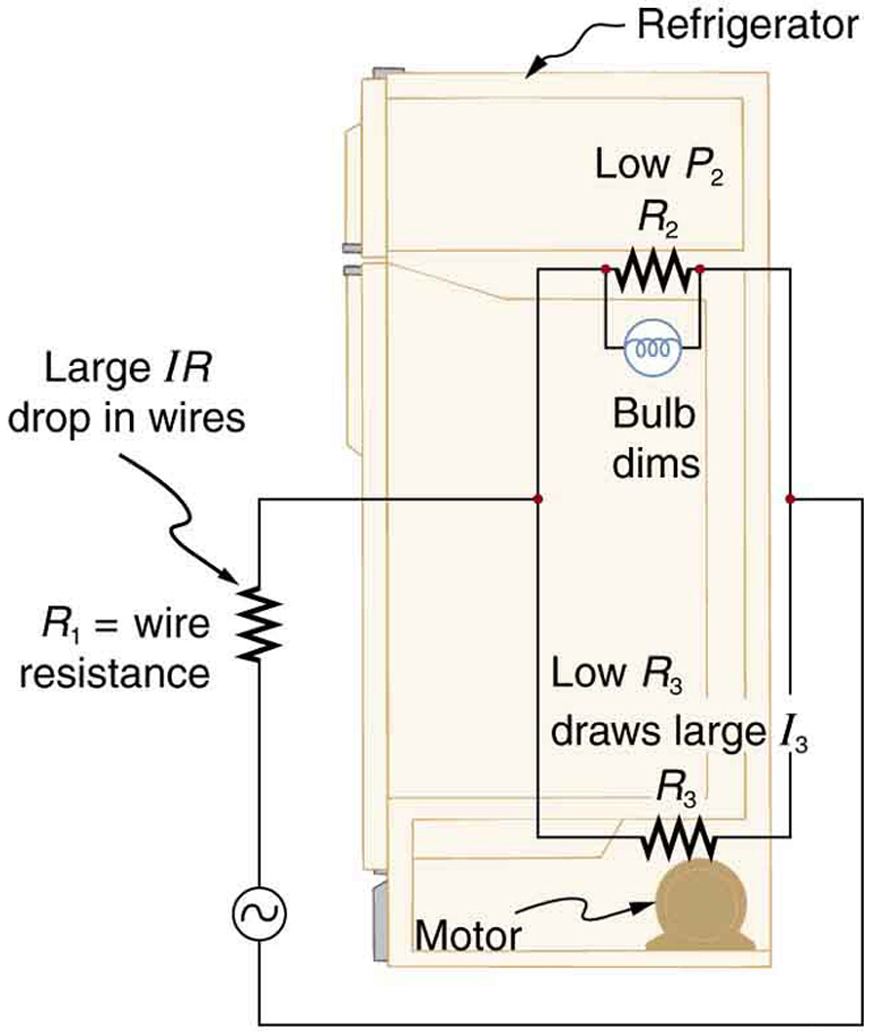Figure 6. Why do lights dim when a large appliance is switched on? The answer is that the large current the appliance motor draws causes a significant IR drop in the wires and reduces the voltage across the light.

1: Can any arbitrary combination of resistors be broken down into series and parallel combinations? See if you can draw a circuit diagram of resistors that cannot be broken down into combinations of series and parallel.

### Problem-Solving Strategies for Series and Parallel Resistors

1. Draw a clear circuit diagram, labeling all resistors and voltage sources. This step includes a list of the knowns for the problem, since they are labeled in your circuit diagram.
2. Identify exactly what needs to be determined in the problem (identify the unknowns). A written list is useful.
3. Determine whether resistors are in series, parallel, or a combination of both series and parallel. Examine the circuit diagram to make this assessment. Resistors are in series if the same current must pass sequentially through them.
4. Use the appropriate list of major features for series or parallel connections to solve for the unknowns. There is one list for series and another for parallel. If your problem has a combination of series and parallel, reduce it in steps by considering individual groups of series or parallel connections, as done in this module and the examples. Special note: When finding Rp , the reciprocal must be taken with care.
5. Check to see whether the answers are reasonable and consistent. Units and numerical results must be reasonable. Total series resistance should be greater, whereas total parallel resistance should be smaller, for example. Power should be greater for the same devices in parallel compared with series, and so on.

# Section Summary

• The total resistance of an electrical circuit with resistors wired in a series is the sum of the individual resistances: Rs = R1 + R2 + R3 + ….
• Each resistor in a series circuit has the same amount of current flowing through it.
• The voltage drop, or power dissipation, across each individual resistor in a series is different, and their combined total adds up to the power source input.
• The total resistance of an electrical circuit with resistors wired in parallel is less than the lowest resistance of any of the components and can be determined using the formula: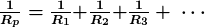• Each resistor in a parallel circuit has the same full voltage of the source applied to it.
• The current flowing through each resistor in a parallel circuit is different, depending on the resistance.
• If a more complex connection of resistors is a combination of series and parallel, it can be reduced to a single equivalent resistance by identifying its various parts as series or parallel, reducing each to its equivalent, and continuing until a single resistance is eventually reached.

### Conceptual Questions

1: A switch has a variable resistance that is nearly zero when closed and extremely large when open, and it is placed in series with the device it controls. Explain the effect the switch in Figure 7 has on current when open and when closed.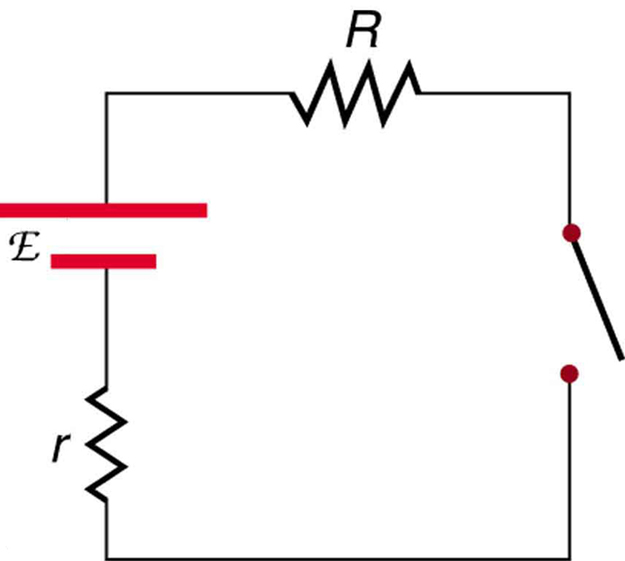Figure 7. A switch is ordinarily in series with a resistance and voltage source. Ideally, the switch has nearly zero resistance when closed but has an extremely large resistance when open. (Note that in this diagram, the script E represents the voltage (or electromotive force) of the battery.)

2: What is the voltage across the open switch in Figure 7?

3: There is a voltage across an open switch, such as in Figure 7. Why, then, is the power dissipated by the open switch small?

4: Why is the power dissipated by a closed switch, such as in Figure 7, small?

5: A student in a physics lab mistakenly wired a light bulb, battery, and switch as shown in Figure 8. Explain why the bulb is on when the switch is open, and off when the switch is closed. (Do not try this—it is hard on the battery!)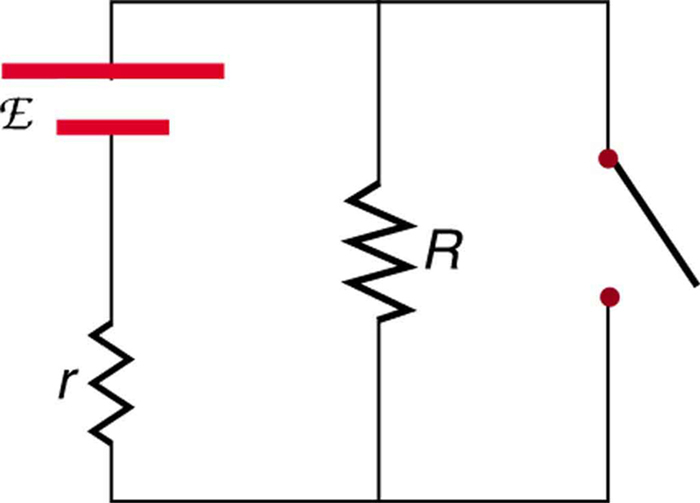Figure 8. A wiring mistake put this switch in parallel with the device represented by R. (Note that in this diagram, the script E represents the voltage (or electromotive force) of the battery.)

6: Knowing that the severity of a shock depends on the magnitude of the current through your body, would you prefer to be in series or parallel with a resistance, such as the heating element of a toaster, if shocked by it? Explain.

7: Would your headlights dim when you start your car’s engine if the wires in your automobile were superconductors? (Do not neglect the battery’s internal resistance.) Explain.

8: Some strings of holiday lights are wired in series to save wiring costs. An old version utilized bulbs that break the electrical connection, like an open switch, when they burn out. If one such bulb burns out, what happens to the others? If such a string operates on 120 V and has 40 identical bulbs, what is the normal operating voltage of each? Newer versions use bulbs that short circuit, like a closed switch, when they burn out. If one such bulb burns out, what happens to the others? If such a string operates on 120 V and has 39 remaining identical bulbs, what is then the operating voltage of each?

9: If two household lightbulbs rated 60 W and 100 W are connected in series to household power, which will be brighter? Explain.

10: Suppose you are doing a physics lab that asks you to put a resistor into a circuit, but all the resistors supplied have a larger resistance than the requested value. How would you connect the available resistances to attempt to get the smaller value asked for?

11: Before World War II, some radios got power through a “resistance cord” that had a significant resistance. Such a resistance cord reduces the voltage to a desired level for the radio’s tubes and the like, and it saves the expense of a transformer. Explain why resistance cords become warm and waste energy when the radio is on.

12: Some light bulbs have three power settings (not including zero), obtained from multiple filaments that are individually switched and wired in parallel. What is the minimum number of filaments needed for three power settings?

### Problem Exercises

Note: Data taken from figures can be assumed to be accurate to three significant digits.

1: (a) What is the resistance of ten 275 Ω resistors connected in series? (b) In parallel?

2: (a) What is the resistance of a 1.00 x102 Ω , a  2.50 kΩ, and a 4.00 kΩ resistor connected in series? (b) In parallel?

3: What are the largest and smallest resistances you can obtain by connecting a 36.0 Ω, a 50.0 Ω, and a 700 Ω resistor together?

4: An 1800-W toaster, a 1400-W electric frying pan, and a 75-W lamp are plugged into the same outlet in a 15-A, 120-V circuit. (The three devices are in parallel when plugged into the same socket.). (a) What current is drawn by each device? (b) Will this combination blow the 15-A fuse?

5: Your car’s 30.0-W headlight and 2.40-kW starter are ordinarily connected in parallel in a 12.0-V system. What power would one headlight and the starter consume if connected in series to a 12.0-V battery? (Neglect any other resistance in the circuit and any change in resistance in the two devices.)

6: (a) Given a 48.0-V battery and  24.0 Ω and 96.0 Ω resistors, find the current and power for each when connected in series. (b) Repeat when the resistances are in parallel.

7: Referring to the example combining series and parallel circuits and Figure 5, calculate Iin the following two different ways: (a) from the known values of I and I2; (b) using Ohm’s law for R3. In both parts explicitly show how you follow the steps in the Problem-Solving Strategies for Series and Parallel Resistors.

8: Referring to Figure 5: (a) Calculate P3 and note how it compares with Pfound in the first two example problems in this module. (b) Find the total power supplied by the source and compare it with the sum of the powers dissipated by the resistors.

11: Show that if two resistors  Rand R2 are combined and one is much greater than the other ( R1 >> R2 ): (a) Their series resistance is very nearly equal to the greater resistance R1. (b) Their parallel resistance is very nearly equal to smaller resistance R2.

12: Unreasonable Results

Two resistors, one having a resistance of 145 Ω, are connected in parallel to produce a total resistance of 150 Ω  (a) What is the value of the second resistance? (b) What is unreasonable about this result? (c) Which assumptions are unreasonable or inconsistent?

13: Unreasonable Results

Two resistors, one having a resistance of  900 kΩ , are connected in series to produce a total resistance of  0.500 MΩ  (a) What is the value of the second resistance? (b) What is unreasonable about this result? (c) Which assumptions are unreasonable or inconsistent?

## Glossary

series
a sequence of resistors or other components wired into a circuit one after the other
resistor
a component that provides resistance to the current flowing through an electrical circuit
resistance
causing a loss of electrical power in a circuit

V = IR

voltage
the electrical potential energy per unit charge; electric pressure created by a power source, such as a battery
voltage drop
the loss of electrical power as a current travels through a resistor, wire or other component
current
the flow of charge through an electric circuit past a given point of measurement
Joule’s law
the relationship between potential electrical power, voltage, and resistance in an electrical circuit, given by: Pe = IV
parallel
the wiring of resistors or other components in an electrical circuit such that each component receives an equal voltage from the power source; often pictured in a ladder-shaped diagram, with each component on a rung of the ladder

### Solutions

1: No, there are many ways to connect resistors that are not combinations of series and parallel, including loops and junctions. In such cases Kirchhoff’s rules, to be introduced in Chapter 21.3 Kirchhoff’s Rules, will allow you to analyze the circuit.

Problems Exercises

1: (a) 2.75 kΩ (b)  27.5 Ω

3: (a) 786 Ω  (b) 20.3 Ω

4: I = P/V Itoaster = 1800 W/120 V = 15.0 A, Ipan = 1400 W/120 V = 11.7 A, Ibulb =75 W/120 V = 0.625 A b) total current = 15.0 A + 11.7A + 0.625 A = 27.3 A so it will blow the 15 A fuse.

5: 29.6 W

7: (a) 0.74 A  (b) 0.742 A

11: (a)  Rs = R1 + R    R_s  ≈ R_1 (R_1 >> R_2)

(b)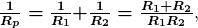so that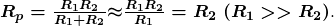13: (a) -400 kΩ . (b) Resistance cannot be negative.

(c) Series resistance is said to be less than one of the resistors, but it must be greater than any of the resistors.